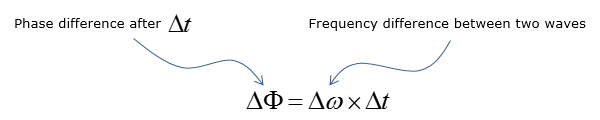Electronics - PLL                                                  Home : www.sharetechnote.com PLL stands for Phase Locked Loop. As the name implies and as shown in the illustration below, PLL is a kind of a circuit with feedback LOOP to keep the phase/frequency of feedbacked signal same (LOCKED) as the phase/frequency of Reference Input Signal.   As shown in the following diagram, if there is phase difference between Reference Input and Feedbacked Input, "Phase detector + Loop Filter" generate a certain level of voltage to change (control) VCO (Voltage Controlled Oscillator).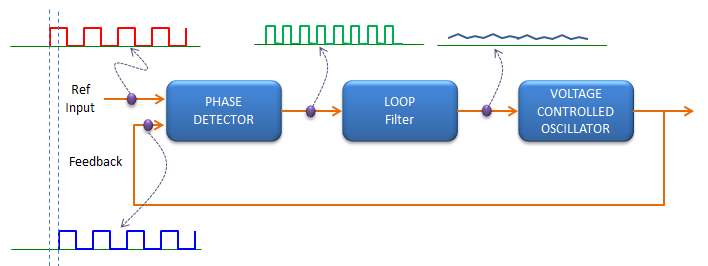Now, getting into a little more details.. let's think about how Phase Detector work. There are a couple of different types of phase detector, but in simplest of form of phase detector work as follows. It just compare two signal level of Reference Input signal and feedback signal every points in time domain, if the both signal is ON, Phase detector genete 'OFF' level (Level 0) and if only one of the signal is ON, the phase detector generate 'ON' level. Simply put, in this case the phase detector is exactly same as XOR Gate logic. Therefore, if the phase of the two input signal is different, Phase detector would generate a terain of pulses. The width of pulse is in proportion to the degree of phase difference. Once these pulses are generated, it goes though a Low Pass Filter (it is called LOOP filter) to smoothen out the pulses to make more flat (DC like) signal. And this DC like signal act as control voltage for VCO.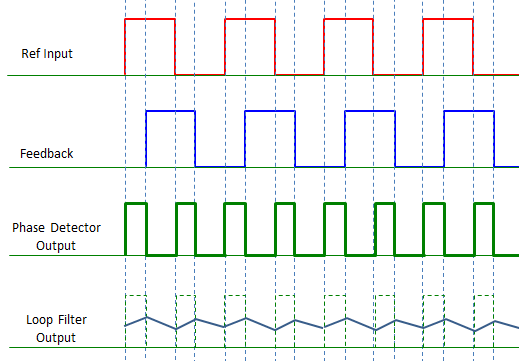Looks not so complicated. But there is an issue with the PLL as explained above. If you simply apply the rule explained above, the phase detector cannot make any difference whether the feedback signal phase is leading or lagging in comparison to reference signal phase as illustrated below.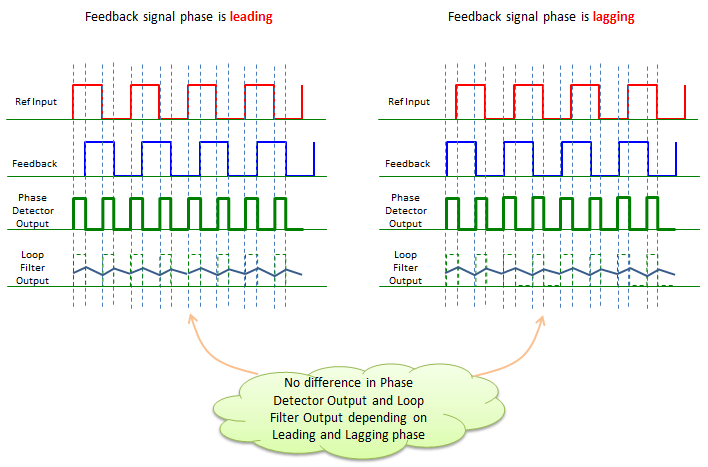So the next step evolution to PLL would be to develop a phase detector that gives the different result depending on whether the feedback signal phase is leading or lagging in comparison to reference signal phase. In this figure, you see that the phase detector generate output signal with different polarity (different sign) depending on whether the phase is leading or lagging. Is there any of PLL which is using this kind of detector ? Of course, there are many of these PLL. (I will explain more about the phase detector of this type later when I have time.)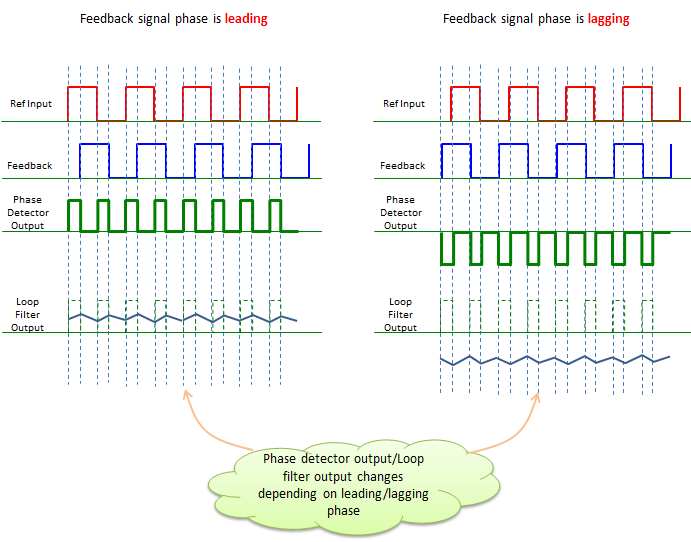Now I think I understand how PLL can detect phase difference and compensate those phase difference. But when I heard that one of the most common application of PLL is frequency synthesize and it implies that it can detect Frequency difference and can lock the output frequency to a specific value. I took me long time to finally understand the correlation between PLL operation and frequency synthesis. The secrete is to put a frequency devider in feedback path as shown below. In this way, we can lock the output frequency of VCO (Voltage Controlled Oscillator) to (N x Ref Input frequency)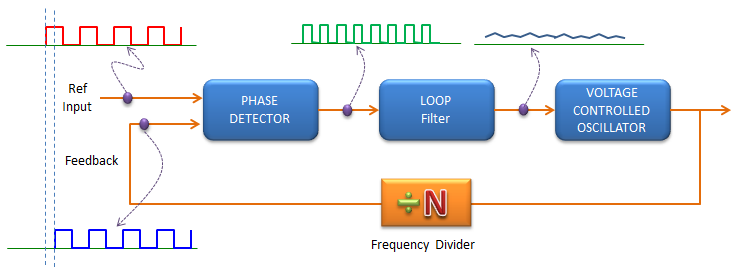For example, if you put '% 2' divider on the feedback path, you can lock the frequency to twice as high as the frequency of Ref Input frequency.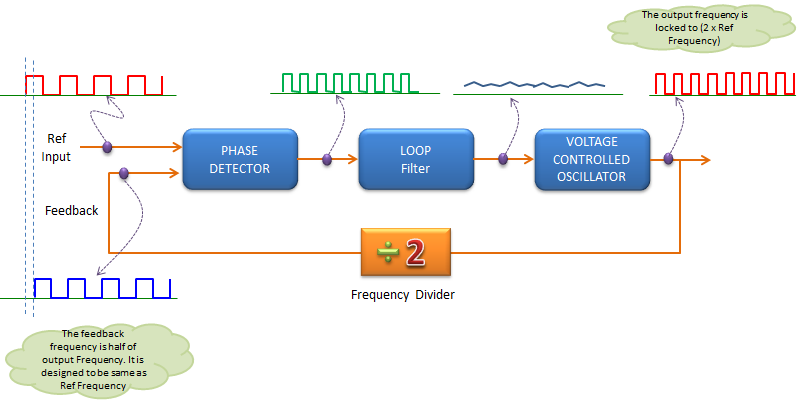How PLL can lock to a specific Frequency ?   As you might have noticed, the critial part of PLL mechanism is to detect Phase difference between a reference signal and input signal. But when we talk about PLL, in most case it would refer to a device that can lock a frequecny of a signal to a specific reference frequency. Then, my question was how the Phase detection mechanism can detect the frequency difference. I found it not easy to visualize the relationship between Phase and frequency, but from following mathmatical equations it can be seen obvious that there is a certain deterministic relationship between phase and frequency.   Following equation shows that Phase accumulated over a certain time span is the result of integration of frequency over the same time period.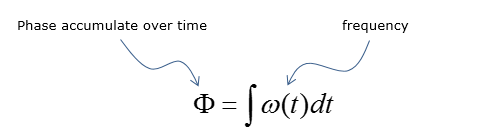Following equation shows that phase difference between two signal (starting with the same phase) after a certain time span.